1. /
2. CBSE
3. /
4. Class 09
5. /
6. Mathematics
7. /
8. NCERT Solutions for Class...

# NCERT Solutions for Class 9 Maths Exercise 3.3### myCBSEguide App

Download the app to get CBSE Sample Papers 2023-24, NCERT Solutions (Revised), Most Important Questions, Previous Year Question Bank, Mock Tests, and Detailed Notes.

NCERT solutions for Class 9 Maths Coordinate Geometry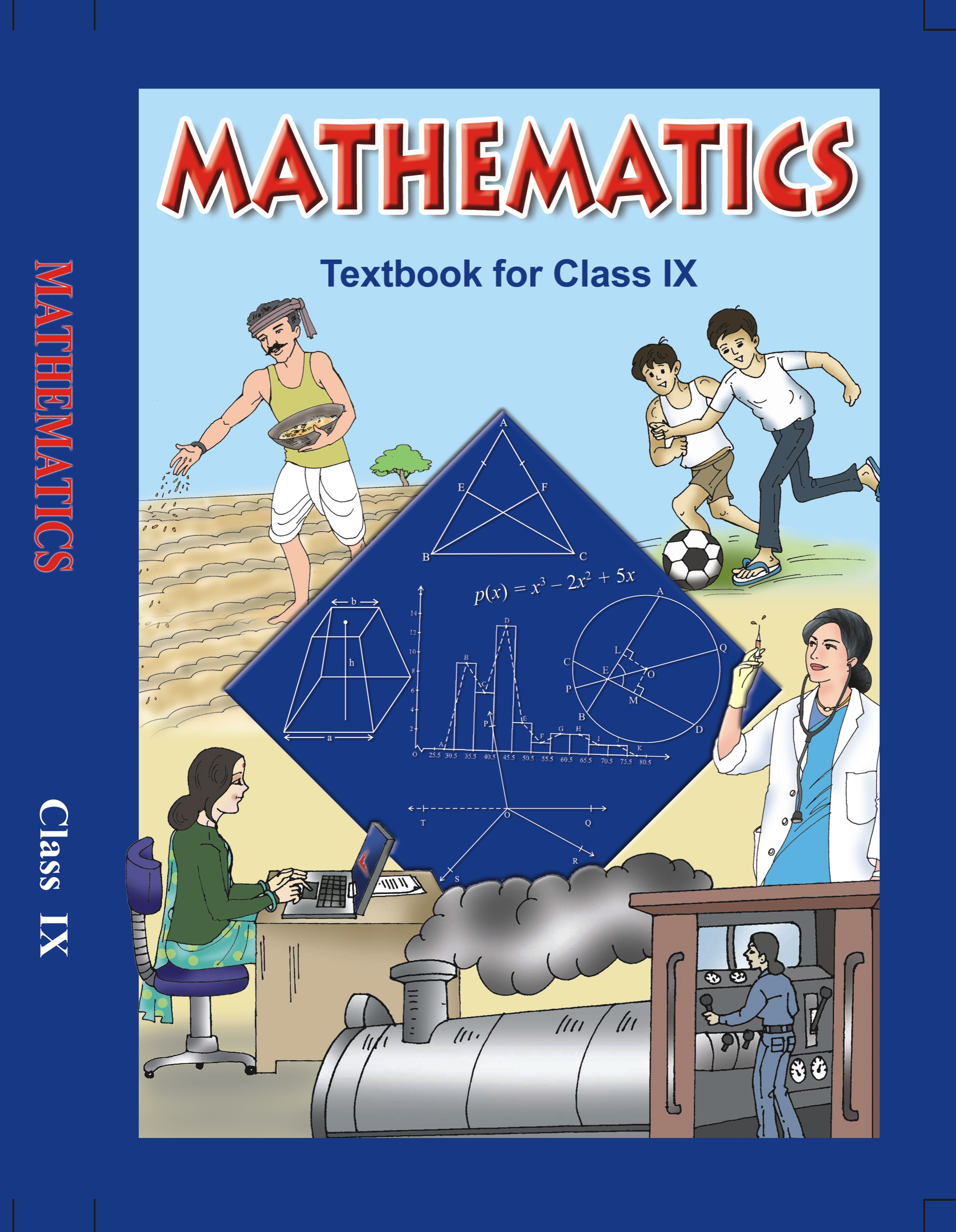## NCERT Solutions for Class 9 Mathematics Coordinate Geometry

###### 1. In which quadrant or on which axis do each of the points (- 2, 4), (3, – 1), (- 1, 0), (1, 2) and (- 3, – 5) lie? Verify your answer by locating them on the Cartesian plane.

Ans. We need to determine the quadrant or axis of the points (2, 4), (3, 1), (- 1, 0), (1, 2) and (3, 5).

First, we need to plot the points (2, 4), (3, 1), (- 1, 0), (1, 2) and (3, 5) on the graph, to get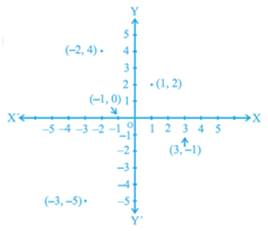We need to determine the quadrant, in which the points (2, 4), (3, 1), (- 1, 0), (1, 2) and (3, 5) lie.

From the figure, we can conclude that the point (2, 4) lie in IInd quadrant.

From the figure, we can conclude that the point (3, 1) lie in IVth quadrant.

From the figure, we can conclude that the point (1, 0) lie on x-axis.

From the figure, we can conclude that the point (1, 2) lie in Ist quadrant.

From the figure, we can conclude that the point (3, 5) lie in IIIrd quadrant.

NCERT Solutions for Class 9 Maths Exercise 3.3

###### 2. Plot the points (x, y) given in the following table on the plane, choosing suitable units of distance on the axes.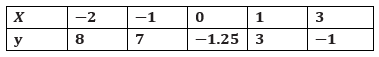Ans. We need to plot the given below points on the graph by using a suitable scale.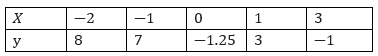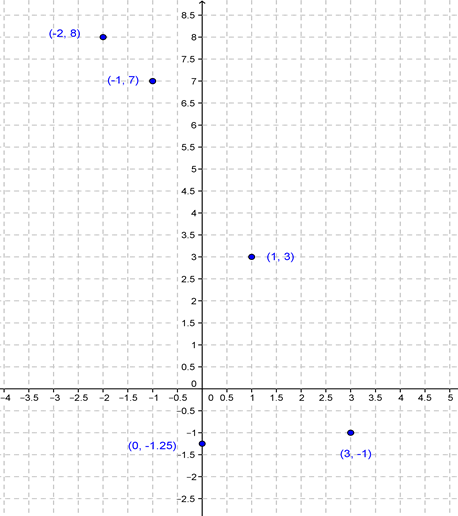## NCERT Solutions for Class 9 Maths Exercise 3.3

NCERT Solutions for Class 9 Maths PDF (Download) Free from myCBSEguide app and myCBSEguide website. Ncert solution class 9 Maths includes text book solutions from Mathematics Book. NCERT Solutions for CBSE Class 9 Maths have total 15 chapters. 9 Maths NCERT Solutions in PDF for free Download on our website. Ncert Maths class 9 solutions PDF and Maths ncert class 9 PDF solutions with latest modifications and as per the latest CBSE syllabus are only available in myCBSEguide.

## CBSE app for Class 9

To download NCERT Solutions for Class 9 Maths, Computer Science, Home Science,Hindi ,English, Social Science do check myCBSEguide app or website. myCBSEguide provides sample papers with solution, test papers for chapter-wise practice, NCERT solutions, NCERT Exemplar solutions, quick revision notes for ready reference, CBSE guess papers and CBSE important question papers. Sample Paper all are made available through the best app for CBSE students and myCBSEguide website.### Test Generator

Create question paper PDF and online tests with your own name & logo in minutes.### myCBSEguide

Question Bank, Mock Tests, Exam Papers, NCERT Solutions, Sample Papers, Notes

1. Maths ex.33